# APPENDIX A TO DECISION NO. 425-R-2011

 This Appendix describes in detail the methodology and the data sources the Agency will use for making cost of capital determinations for federally-regulated railway companies. Cost of capital determinations are made annually for CN and CP, and as required for other railway companies in regulatory cost proceedings before the Agency.

 For CN and CP, the Agency will make three separate cost of capital determinations annually:

1. Cost of capital rate for the transportation of western grain, to be used in the composite price index calculation under subsection 151(1) of the Canada Transportation Act;
2. Cost of capital rate for the development of interswitching costs and rates; and,
3. Cost of capital rate to be used for regulatory purposes other than for calculating the western grain composite price index and establishing regulated interswitching rates.

 With the exception of the risk-free rate of return defined below, all of the elements necessary for the development of all three cost of capital rates will be those determined annually in the cost of capital rate for the transportation of western grain.

## Asset base

 The asset base will be the net rail investment. Net rail investment is defined as the gross book value of all railway assets less accumulated depreciation, and represents the portion of the railway company's net assets that are providing railway transportation services and are under Agency jurisdiction. The net rail investment includes an amount for working capital. Net rail investment will be determined using book values. Based on their most recent year-end financial statements, each railway company will make a submission with respect to their net rail investment, which will be verified by the Agency.

## Capital structure

 The capital structure describes the proportions of the various sources of capital used to finance the net rail investment, and will be comprised of long-term debt, shareholders equity and deferred taxes. Capital structure will be determined using book values. Based on their most recent year-end financial statements, each railway company will make a submission with respect to their capital structure, which will be verified by the Agency.

## Cost rate of debt

 The cost rate of long-term debt will be the weighted rates of interest or premiums actually paid by the company on its debt instruments, as recorded in each railway company's most recent year-end financial statements and submitted to the Agency. These submissions will be verified by the Agency, prior to its determination of each railway company's cost rate of long-term debt.

## Cost rate of deferred taxes

 Deferred taxes will be allocated a zero cost rate, as they represent a source of capital for which there are no interest premiums or shareholder obligations payable.

## Cost rate of common equity

 The cost rate of common equity for each railway company will be determined separately for the Canadian and U.S. markets using the Capital Asset Pricing Model (CAPM).

 The CAPM is defined as: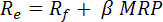Text alternative for CAPM equation

where:

Re
is the cost rate of common equity;
Rf
is the risk-free rate of return;
MRP
is the historical market risk premium or excess stock market return over the risk-free rate; and
β
is the measure of undiversifiable risk associated with the railway company's stock returns.

 The Canadian cost rate of common equity will be determined by calculating a single cost rate of common equity using Government of Canada 3-5 year bond yields for the risk-free rate of return. The U.S. cost rate of common equity will be determined by calculating two distinct cost rates of common equity, one using 3-year U.S. Treasury bond yields for the risk-free rate of return, and the other using 5-year U.S. Treasury bond yields for the risk-free rate of return, and taking an averageFootnote 1 of the two rates.

 The methodology for determining each of the components of the CAPM is specified below.

### Risk-free rate

 For the U.S. cost rate of common equity, two distinct risk-free rates will be determined using the yields on U.S. 3-year and 5-year Treasury bonds.Footnote 3 For determining the cost of capital rate for the transportation of western grain, the values used will be the average of daily yield observations for the month of January on each of 3-year and 5-year U.S. Treasury bonds, as published by the Federal Reserve. For the cost of capital for the development of interswitching costs and rates, the values used will be the average of daily yield observations for the month of September, on each of 3-year and 5-year U.S. Treasury bonds, as published by the Federal Reserve. For the cost of capital rate for other regulatory purposes, the values used will be the average yields for the calendar year for which the determination is being made, on each of 3-year and 5-year U.S. Treasury bonds, as published by the Federal Reserve.

 The market risk premium is the arithmetic average of annual excess returns of the stock market over the risk-free rate, as measured from the year 1951 to the present for the Canadian cost rate of common equity, and from the year 1954 to the present for the U.S. cost rate of common equity. It will be estimated using the equation:Text alternative for MRP equation

where:

Rm,t
is the market return in year t. For the Canadian cost rate of common equity, this value is derived as the percentage change in the closing value of the S&P/TSX Composite Total Return IndexFootnote 4 in year t over the closing value of the same market index in year t-1. For the U.S. cost rate of common equity, this value is derived as the percentage change in the closing value of the S&P 500 Composite Total Return IndexFootnote 5  in year t over the closing value of the same market index in year t-1.

Each market return in year t will be estimated using the equation:Text alternative for Market Return equation

where:

Im,t
is the closing stock market index value in year t; and
Im,t-1
is the closing stock market index value in year t-1
Rf,t
is the risk-free return in year t. For the Canadian cost rate of common equity, this will be estimated as the month of January income return on Government of Canada 3-5 year marketable bondsFootnote 6 in year t. For the U.S. cost rate of common equity, two sets of risk-free returns comprising the month of January income returns on each of U.S. 3-year and 5-year Treasury bondsFootnote 7 , will be estimated for each year t
T
represents the number of years for which reliable market data on market returns and bond yields are available, and will comprise the years from 1951 to the present for the Canadian cost rate of common equity, and 1954 to the present for the U.S. cost rate of common equity.

### Beta

 Beta for each railway company will be estimated using the most recent five years of weekly dataFootnote 8  in the regression equation:Text alternative for the equation

where:

Rc,w
for the Canadian cost rate of common equity is the weekly return on the stock of company c on the Toronto Stock Exchange in week wFootnote 9 , derived as the percentage change in the company's stock price in week w; and for the U.S. cost rate of common equity is the weekly return on the stock of company c on the New York Stock Exchange in week wFootnote 10 , derived as the percentage change in the company's stock price in week w.

Each weekly observation is estimated as:Text alternative for the equation

where:

Pc,w
is the closing price of company c in week w;
Pc,w-1
is the closing price of company c in week w-1.
Rf,w
for the Canadian cost rate of common equity is the weekly income return on Government of Canada 3-month marketable bondsFootnote 11 , and for the U.S. cost rate of common equity is the weekly income return on U.S. 3-month Treasury bondsFootnote 12 ;
Rm,w
for the Canadian cost rate of common equity is the weekly return on the S&P/TSX Composite Index, derived as the percentage change in the closing value of the S&P/TSX Composite IndexFootnote 13 in week w over the closing value of the same market index in week w-1, and for the U.S. cost rate of common equity is the weekly return on the S&P 500 Index, derived as the percentage change in the closing value of the S&P 500 IndexFootnote 14 > in week w over the closing value of the same market index in week w-1.

Each market return observation will be estimated using the equation:Text alternative for the equation

where:

Im,w
is the closing stock market index value in week w; and
Im,w-1
is the closing stock market index value in week w-1.
αc
is a regression parameter using Ordinary Least Squares (OLS) regression;
βc
is the company beta and is a regression parameter in the (OLS) regression; and
e
is a stochastic error term.

 Company betasFootnote 15 will be adjusted for mean-reversion using the Blume adjustment procedure, which is applied as: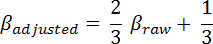Text alternative for the equation

where:

is the adjusted company beta to be used in the cost rate of common equity calculation; and
βraw
is the company beta obtained from the regression analysis.

### Canada/U.S. cost rate of common equity

 The Canada/U.S. cost rate of common equity will be the weighted average of the Canadian and U.S. cost rates of common equity, with the weights being, for each railway company, based on the volume of shares traded on the Toronto and New York stock exchanges, respectivelyFootnote 16 . Weights will be determined as the relative proportions of the daily trading volumes of each company on the Toronto and New York stock exchanges during the most recently ended calendar year. Specifically, the Canada/U.S. cost rate of common equity is to be estimated as: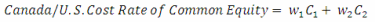Text alternative for the equation

where:

C1
is the Canadian cost rate of common equity;
C2
is the U.S. cost rate of common equity; and
VT,d
is the volume traded on the Toronto Stock Exchange on day d, for each day of the most recently ended calendar year;
VN,d
is the volume traded on the New York Stock Exchange on day d, for each day of the most recently ended calendar year; and
n
is the number of trading days in the most recently ended calendar year.

## Tax adjusted cost rate of common equity

 An income tax allowance, based on the railway companies' submitted Canadian statutory federal and provincial income tax rate, will be added to the Canada/U.S. cost rate of common equity to adjust the value of the Canada/U.S. cost rate of common equity to a before-tax basis. No income tax allowance will be applied to the cost rate of debt as it is income tax deductible.

 The tax adjusted cost rate of common equity for each railway company will be determined using the formulaFootnote 17 :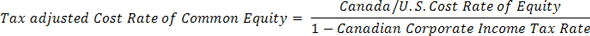Text alternative for the equation

## Weighted average cost of capital

 The proportion of each type of funding in the capital structure will be used to weight each cost rate, the sum of which will be the weighted average cost of capital (WACC) expressed in percentage terms. It will be determined using the formula: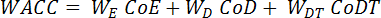Text alternative for the equation

where:

WE
is the proportion of equity in the book value of net rail investment;
CoE
is the tax adjusted cost rate of common equity determined as described above;
WD
is the proportion of long-term debt in the book value of net rail investment;
CoD
is the cost rate of long-term debt determined as described above;
WDT
is the proportion of deferred taxes in the book value of net rail investment; and
CoDT
is the cost rate of deferred taxes, which will be set to a value of zero (0).
Date modified: